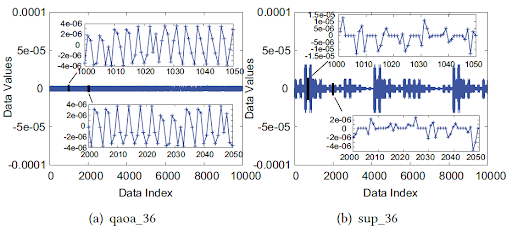# EPiQC Researchers Simulate 61-Bit Quantum Computer With Data Compression

January 13, 2020

When trying to debug quantum hardware and software by using a quantum simulator, every quantum bit (qubit) counts. Every simulated qubit closer to physical machine sizes halves the gap in compute power between the simulation and the physical hardware. However, the memory requirement of full-state simulation grows exponentially with the number of simulated qubits, and this limits the size of simulations that can be run.

Researchers at the University of Chicago and Argonne National Laboratory significantly reduced this gap by using data compression techniques to fit a 61-qubit simulation of Grover’s quantum search algorithm on a large supercomputer with 0.4 percent error. Other quantum algorithms were also simulated with substantially more qubits and quantum gates than previous efforts.

Classical simulation of quantum circuits is crucial for better understanding the operations and behaviors of quantum computation. However, today’s practical full-state simulation limit is 48 qubits, because the number of quantum state amplitudes required for the full simulation increases exponentially with the number of qubits, making physical memory the limiting factor. Given n qubits, scientists need 2^n amplitudes to describe the quantum system.

There are already several existing techniques that trade execution time for memory space. For different purposes, people choose different simulation techniques. This work provides one more option in the set of tools to scale quantum circuit simulation, applying lossless and lossy data compression techniques to the state vectors.

Figure 1 shows an overview of our simulation design. The Message Passing Interface (MPI) is used to execute the simulation in parallel. Assuming we simulate n-qubit systems and have r ranks in total, the state vector is divided equally on r ranks, and each partial state vector is divided into nb blocks on each rank. Each block is stored in a compressed format in the memory.

Figure 2 shows the amplitude distribution in two different benchmarks. “If the state amplitude distribution is uniform, we can easily get a high compression ratio with the lossless compression algorithm,” said researcher Xin-Chuan Wu. “If we cannot get a nice compression ratio, our simulation procedure will adopt error-bounded lossy compression to trade simulation accuracy for compression ratio.”Figure 2. Value changes of quantum circuit simulation data. (a) The data value changes within a range. (b) The data exhibit a high spikiness and variance such that lossless compressors cannot work effectively.

The entire full-state simulation framework with data compression leverages MPI to communicate between computation nodes. The simulation was performed on the Theta supercomputer at Argonne National Laboratory. Theta consists of 4,392 nodes, each node containing a 64-core Intel®Xeon PhiTM processor 7230 with 16 gigabytes of high-bandwidth in-package memory (MCDRAM) and 192 GB of DDR4 RAM.

The full paper, “Full-State Quantum Circuit Simulation by Using Data Compression,” was published by The International Conference for High Performance Computing, Networking, Storage, and Analysis (SC’19). The UChicago research group is from the EPiQC (Enabling Practical-scale Quantum Computation) collaboration, an NSF Expedition in Computing, under grants CCF-1730449. EPiQC aims to bridge the gap from existing theoretical algorithms to practical quantum computing architectures on near-term devices.